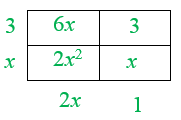Home > INT2 > Chapter 2 > Lesson 2.3.3 > Problem2-106

2-106.

Draw an area model for each expression. Then write an equivalence statement for the area as a product and as a sum. Homework Help ✎

1. $(x+3)(2x+1)$

Remember, each term in the expression is placed on the outside of the box.Be sure to include the diagram above. Here is the equivalence statement:

$2x^2+7x+3$

2. $2x(x+5)$

Refer to hint (a) when drawing the area model.

3. $x(2x+y)$

$2x^2+xy$

4. $(2x+5)(x+y+2)$

$2x^2+2xy+9x+5y+10$